Este curso foi revisado!

Para uma experiência de aprendizado mais agradável, recomendamos que você estude a versão republicada amigável deste curso.

Leve-me ao curso revisado.

- or -

Continue studying this course

• Nota de Estudos
• Rever Tópicos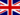Benjamin G. 2 0 you made an error at the end of the video where you introduced F=m x a originaly you had a mass of 70kg which gave you 686 newtons but when you mentioned jumping off of a building you had a mass of 68kg but still had the same newtons..Benjamin G. 1 0 I am struggling to work out how you got 9.8m/s2 from G x mass of the earth divided by the distance... Mass of the earth is 5.9736x10^24kg the distance between the crust and center of the earth is 4.0577294x10^12 meters... how the hell is 6.674x10^-11 x 5.9736x10^24 / 4.0577294x10^12 = 9.8m/s2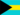Dezonike D. 0 0 What is gravity?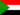Mohammed H. 1 0 gretLee G. 0 0 Explained well and understandable when going through calculation stages.Karen W. 0 0 Clearly described and understandableMail P. 0 0 is gravity pulling (Newton) or is it pushing (Einstein) our mass (you and I) to the earths mass? these two terms pulling and pushing are being used confusingly in physics to define the force, so could it be both, since both masses are acting on the other?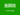Mohammed Muntekhab A. 1 0 How to get the gravitational force = 9.8 m/s2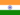MOHIT R. 0 0 Basic but nicely explained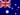Allan R. 0 0 Very well explainedVocê recebeu uma nova notificação

Clique aqui para visualizar todos eles